# Random Variable Algebra Simulation and Intuition

Random Variable Algebra can be challenging to understand mathematically and can sometimes feel counterintuitive. Things that have helped me are learning the wrong ways to perform RV Algebra as well as numerical simulation, both of which are presented in the notebook below.

A recording of me talking over slides is available on Youtube. You can download the notebook from Github

Happy Learning!

# Random Variable Algebra¶

## An applied example with sampling¶

### A bunch of wrong ways, one sort of right way, compared to the always right way¶

But enough to understand why its important and how to build an intuition

# Random variable math can be hard to understand¶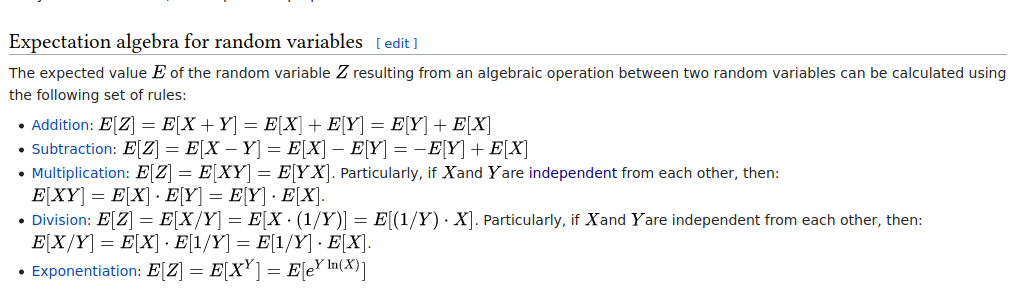# Even Wikipedia knows this¶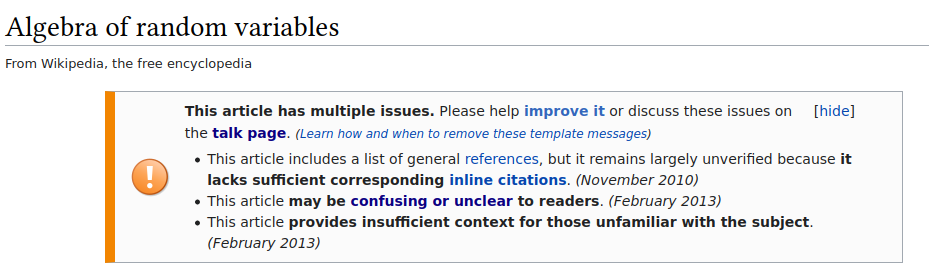# The concepts are relevant to every business and data person out there¶

Too often they ignored or misapplied however. We're going to build intuition with an example and simulation to help clear things up

### The math is important, we're just not covering it here¶

Other people have already done a great job.

If you're working with random variables often I encourage you to learn the formalisms. Great resources are linked below

# What volumes of vaccine do you need to vaccinate 1 million people?¶

• Assume 1 dose is enough
• Each dose is .3ml
• Ignore the vial size constraint for our purposes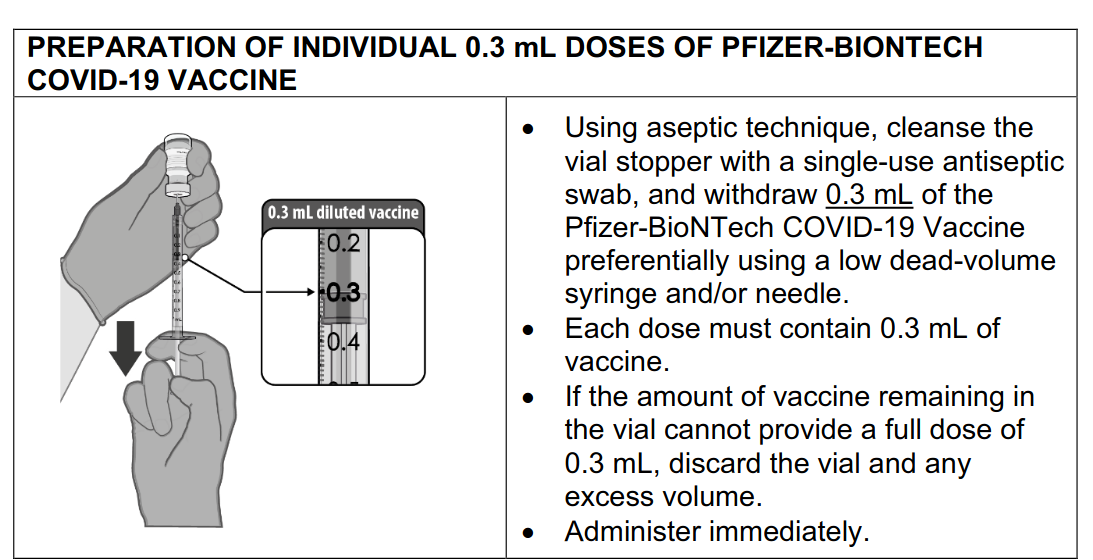## Algebra 101¶

Each dose is .3 ml, for 1 million people we'll need 300,000 ml

If our variable $s$ is size of scoop, and $c$ as number of customers

$$dose = .3 \\ people = 1000000 \\ dose*people = 300000$$

# Algebra 101 (with the help of a computer)¶

In :
number_of_people = 1e6
dose_per_person_ml = .3
number_of_people * dose_per_person_ml

Out:
300000.0

# Unfortunately everything is random¶

Each dose isn't perfectly .3 ml, but we need to define the randomness somehow to work with it

# Defining randomness with a random variable¶

We'll assume the dose is a Gaussian distributed random variable dist. Gaussian distributed is just one of many possible choices, and is a human decision, not a fundamental fact

Since we picked definition means while each observation is random, the relative probability of expected outcomes occurrence is precisely defined

$$\mathcal{N}(\mu = .3, \sigma=.05)$$
In :
from scipy import stats

mean_dose = .3
std_dose = .05
dose_rv = stats.norm(mean_dose, std_dose)
dose_rv.rvs()

Out:
0.2725595065696113
In :
num_random_draws = 10000
samples = dose_rv.rvs(num_random_draws)

fig, ax = plt.subplots()
az.plot_dist(samples, ax=ax)
plt.show()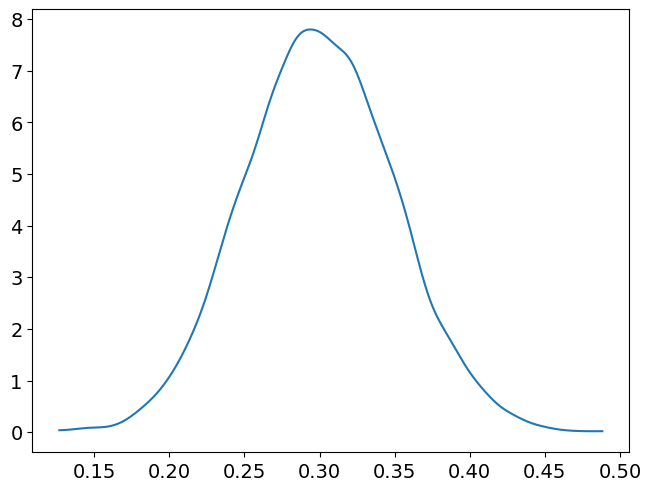# Using simulation (to recover parameters)¶

Because we've computationally defined our RV we can sample from it and make estimations from those samples

In :
num_random_draws = 10000
samples = dose_rv.rvs(num_random_draws)
(f"The recovered mean is {samples.mean()}, "
f"The recovered standard deviation is {samples.std()}")

Out:
'The recovered mean is 0.2997408994886067, The recovered standard deviation is 0.049557663708513'

# Back to the point: How much do we need to vaccinate a million people?¶

We've specified the random variable individual dose but still need to figure out random variable represents a million doses.

But we can just multiply our dose random variable right? right?????

# Wrong Way 1: Multiply the mean and standard deviation by million (because that seems to make sense)¶

This one assumes the mean and standard linearly. The intuitiveness and ease is tempting but with statistics intuition like this is more often wrong than right.

In :
mean = mean_dose*1_000_000
sd = std_dose*1_000_000
mean, sd

Out:
(300000.0, 50000.0)

In this case it happens to be right for the the mean but wrong for the standard deviation

# Wrong Way 2: Multiply a single sample by a million¶

This first dose is random, the next 2,999,999 are then assumed to exactly the same. Besides with just one number how do we calculation the standard deviation?

In :
dose_rv.rvs(1)*1_000_000

Out:
array([309076.79928766])

# Wrong Way 3: Take a million draws and sum them together¶

Each single sample is random which is great but like Wrong Way 2, how would we estimate the uncertainty, which was why we started down this path

In :
samples = dose_rv.rvs(1_000_000)
samples.sum()

Out:
299985.82573344326

# Correctly simulating the entire random process a bunch¶

Simulate the entire sequence of random events not just once, but many many times, then make an estimation from that.

In :
# Decide on the number of simulations
num_trials = 10000

amount_for_million_doses = np.zeros(num_trials)
for i in range(num_trials):

# Take a million random draws once per simulation
amount = dose_rv.rvs(1_000_000).sum()

# Keep track of the simulation result
amount_for_million_doses[i] = amount

In :
(f"The recovered mean is {amount_for_million_doses.mean()}, "
f"The recovered standard deviation is {amount_for_million_doses.std()}")

Out:
'The recovered mean is 299999.65053315327, The recovered standard deviation is 50.71133054352981'

# Comparison to the analytical answer¶

If we know the formula the analytic answer is easier, and more correct

In :
np.sqrt((std_dose**2)*1_000_000)

Out:
50.00000000000001

But I wouldn't blame you if it wasn't "super obvious"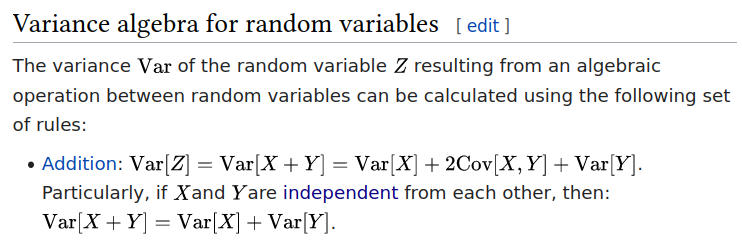# Gaps with simulation¶

• Simulation is not exact and not guaranteed to be close
• How much is "enough" isn't an exact science
• Won't have a fundamental understanding
• Knowing the math is like learning the foundation and the building blocks
• Need to triple check your code
• Subtle mistakes can really mess up the calculations

# In Practice: Check if you need RVs (and avoid them if you can)¶

If you can get away with not using random variables do it, but don't blindly assume you can for every problem

# If Learning: Learn as much math as you can, use simulations to help¶

Use simulation to build intuition and reinforce your knowledge Use simulations if you're up against a deadline and stuck